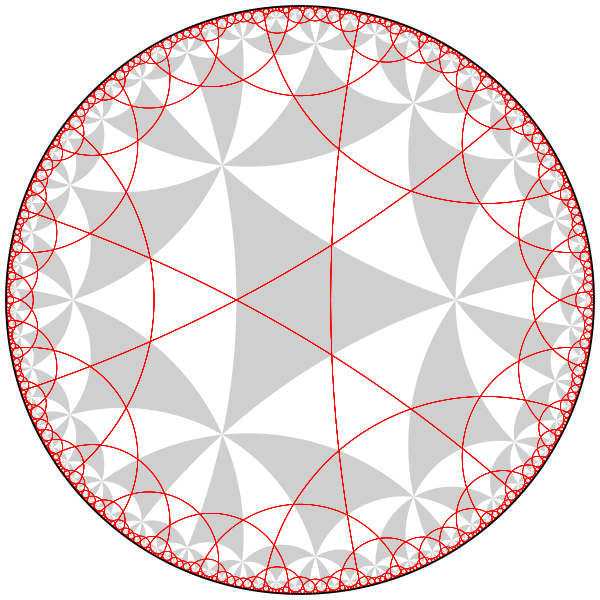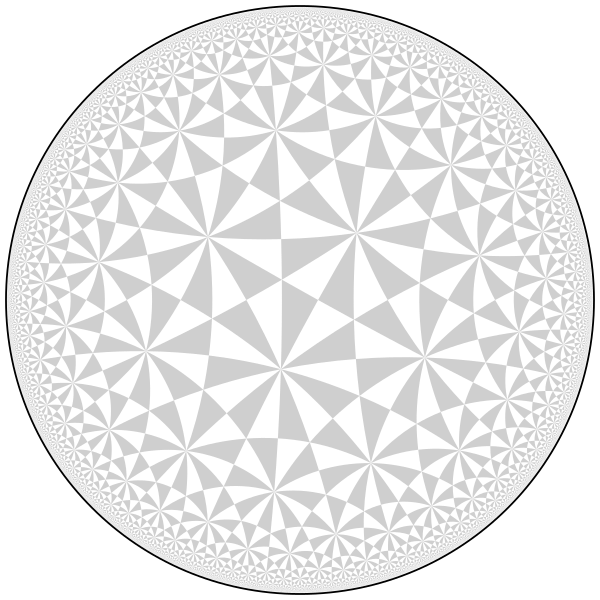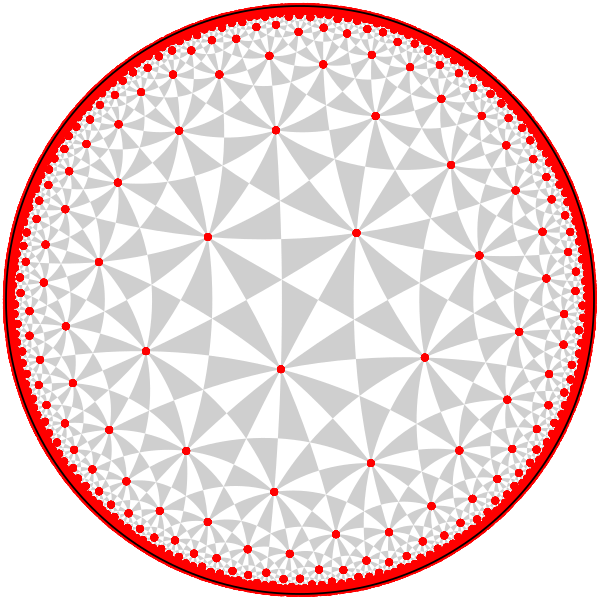draw triangle tilings of the hyperbolic plane
You cannot select more than 25 topics Topics must start with a letter or number, can include dashes ('-') and can be up to 35 characters long.Florian Stecker 1b1880fe19

# hyperbolic tilings

A program to draw regular tilings of the hyperbolic plane by triangles as SVG files.

## Setup

GCC and GNU make are needed. To compile, just run `make`.

## Usage

The executable is called `hyperbolic` and is invoked as follows:

``````\$ ./hyperbolic
Usage: ./hyperbolic <p> <q> <r> <n_elements> <word1> <word2> ...
``````

The arguments `p,q,r` specify the orders of the rotations `bc`, `ca` and `ab`, where `a,b,c` are the reflections along the three sides of the triangle. The parameter `n_elements` limits how many translates of the triangle are being drawn (1000 is a good default value, although for some values of `p,q,r` more are needed).

The program can not only draw the tiling by triangles, but also the axes of specific hyperbolic elements in the triangle group or the fixed points of elliptic elements. To use this feature, just specify the group elements as additional arguments, written as words in the three reflections `a,b,c`. This will draw its axis/fixed point and that of all of its conjugates.

## Examples

``````./hyperbolic 5 5 5 5000 abc
````````````./hyperbolic 2 3 7 20000
````````````./hyperbolic 2 3 7 20000 ab
``````## Conversion to PDF or PNG

If a different image format is required, the SVG files can easily be converted. Using rsvg-convert gives good results.

To convert to PNG (used for the images above):

``````rsvg-convert --format=png -w 600 -h 600 tiling_555_abc.svg -o tiling_555_abc.png
``````

To convert to PDF:

``````rsvg-convert --format=pdf -w tiling_555_abc.svg -o tiling_555_abc.pdf
``````

`maketiling.sh` is a convenience script which takes the same arguments as `hyperbolic`, but saves the SVG file in the folder `output/` (which has to be present) with a descriptive name and also converts it to PDF.